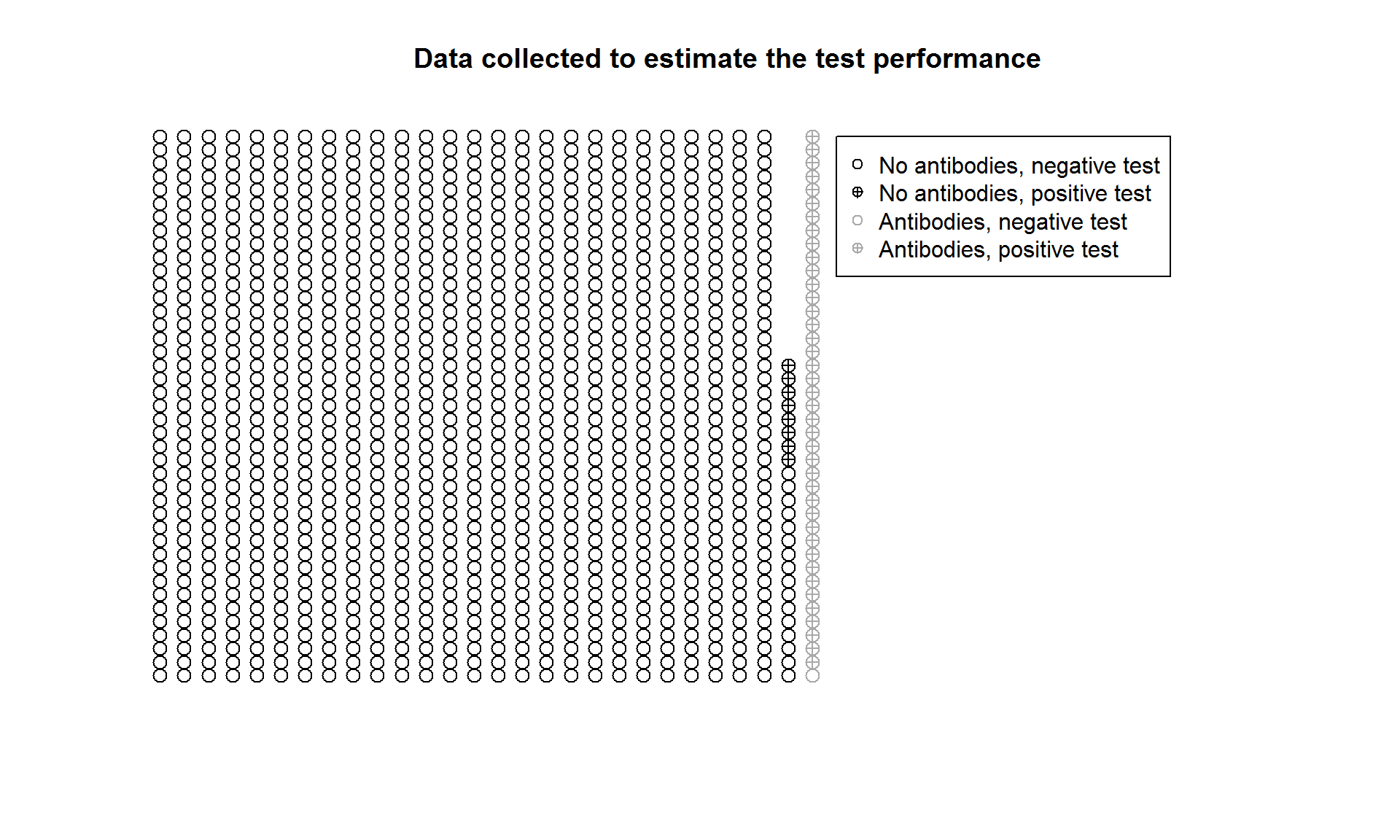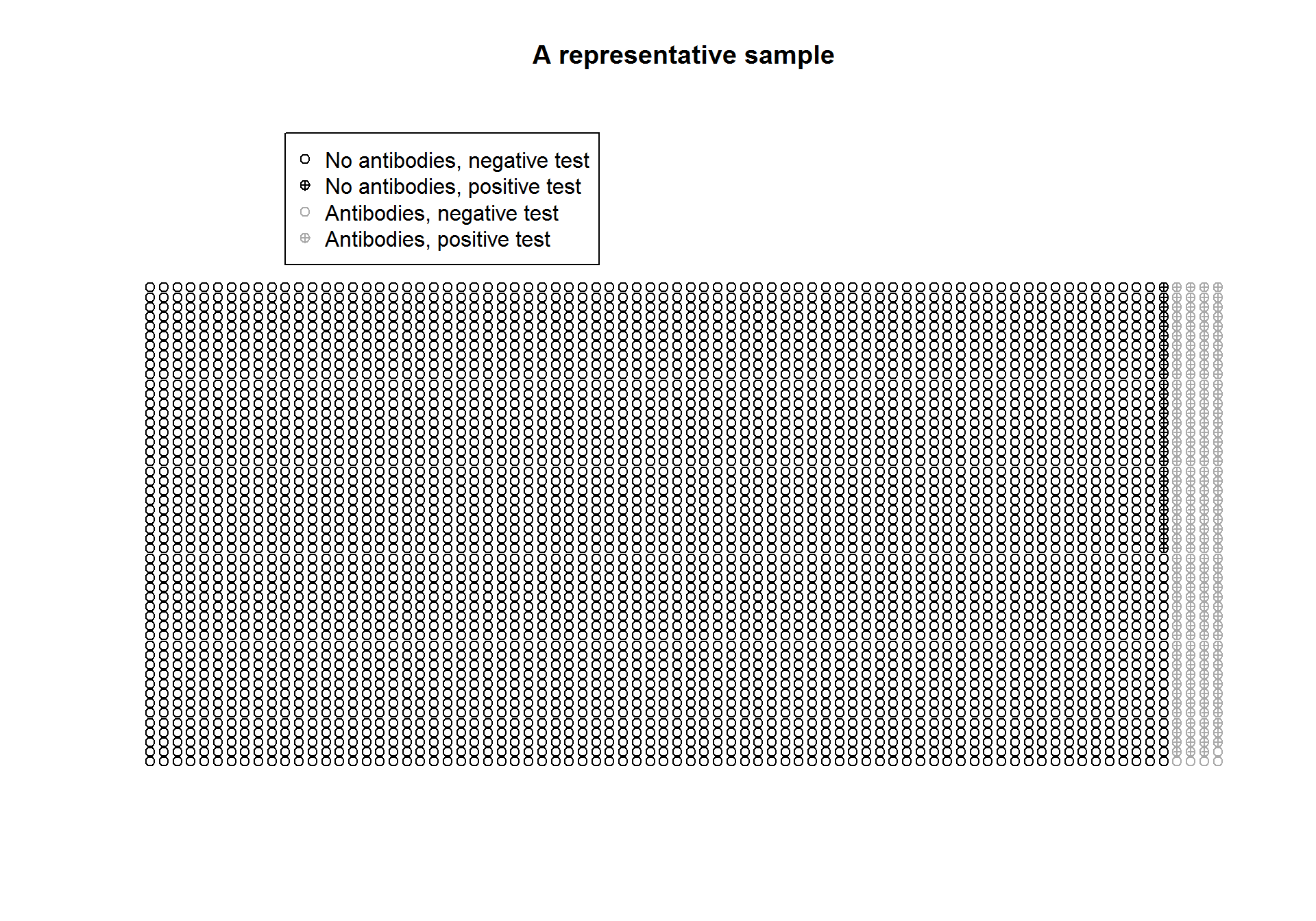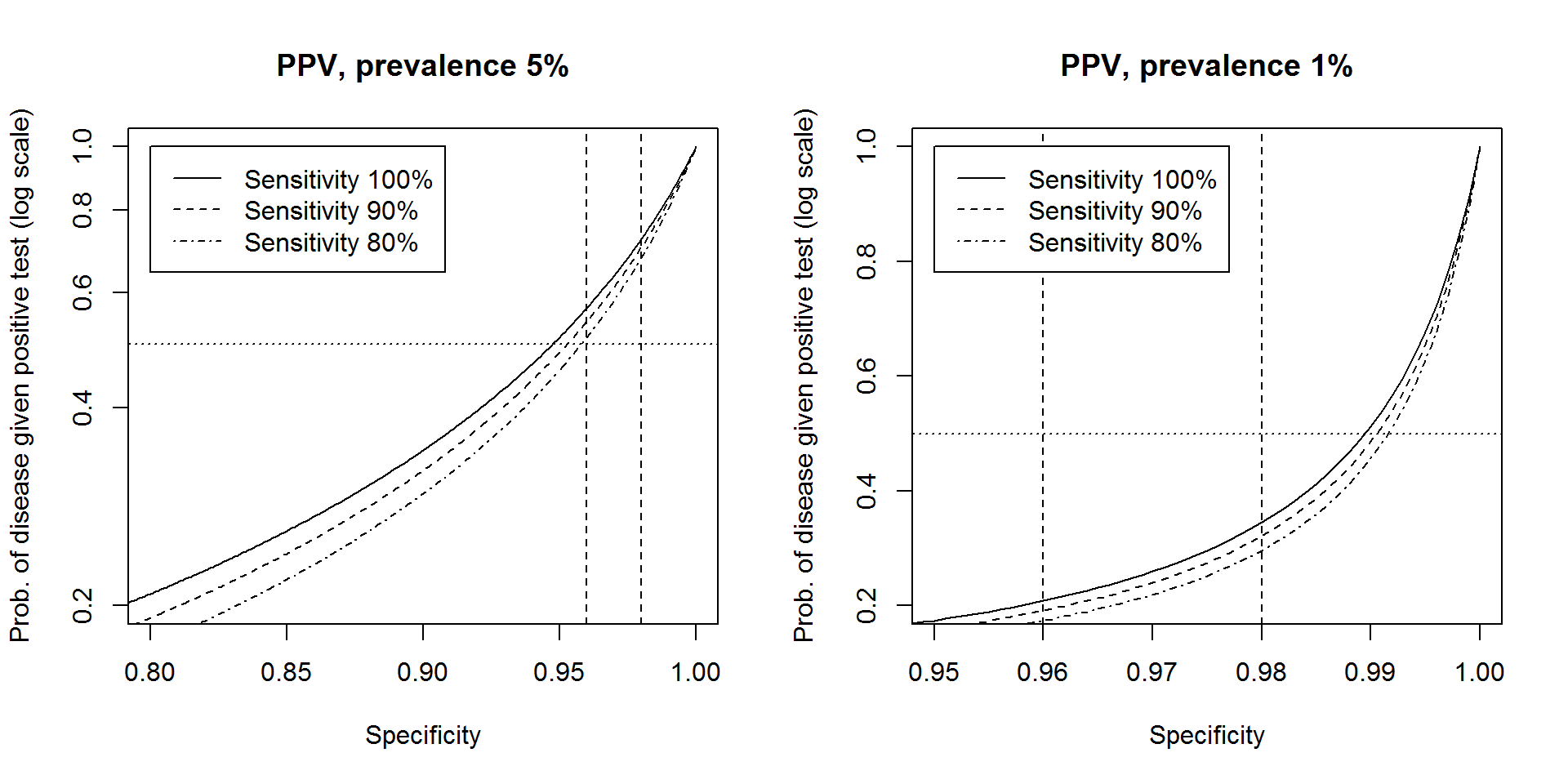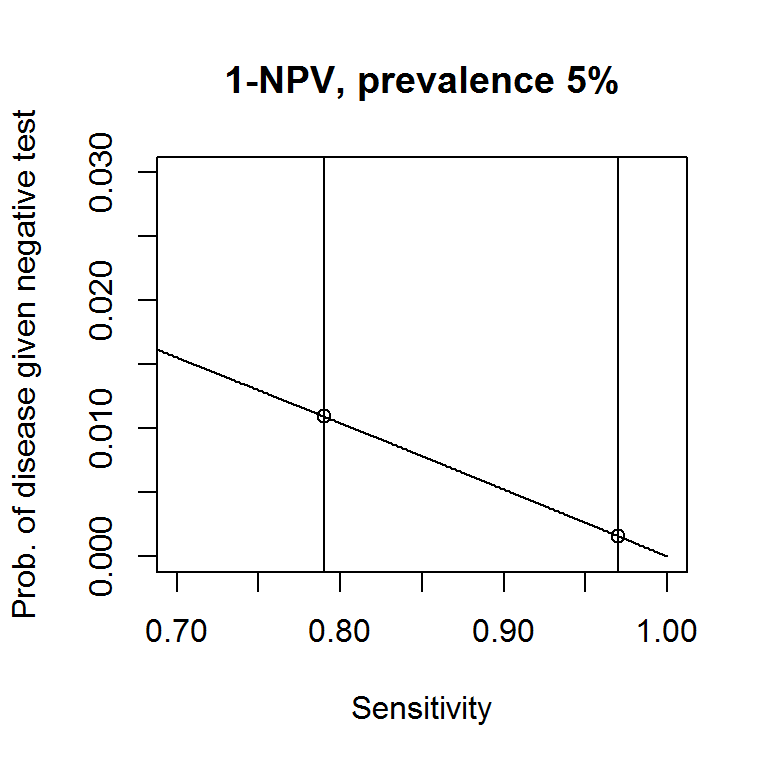# 1 Introduction

On May 13, 2020, the Instituto de Salud Carlos III published a report on the first round of the national epidemiologic study on the infection caused by the SARS-COV-2 (ENE-Covid19). The report contains interesting results and is very clearly written. These notes have been prepared to help you reading and understanding it together with some other epidemiological and statistical studies about the coronavirus COVID-19 pandemic. No basic prior statistical knowledge is required, but having followed an undergraduate Introductory Statistics course would be of some help.

# 2 Biomedical screening tests and associated jargon

The terms prevalence, sensitivity, and specificity are stardard ones in the jargon of Biomedical Sciences.

• The prevalence is the percentage (or porportion) of individuals in a population with some given medical condition.
• Example 1: The current prevalence of IgG antibodies against SARS-Cov2 in Spain is estimated to be $$5\%$$ (ENE-Covid19).
• The sensitivity (True Positive Rate, TPR or Positive Percent Agreement, PPA) is the proportion of infected individuals that are identified as such.
• The specificity (True Negative Rate, TNR or Negative Percent Agreement, NPA) is the proportion of healthy individuals that are identified as such.

In the chart below, you can find a black circumference for each screening test run on an individual withouth antibodies in Example 3, and a grey one for each screening test run on an individual carrying antibodies in Example 2. If the result of the test was positive, there is a ‘+’ inside the circumference.The chart above is not a representative one for the Spanish population, since there are 3.63% grey circumferences (representing individuals with antibodies), while the percentage of Spanish individuals with antibodies is roughly $$5\%$$, see Example 1.

Below, you can find a new chart at which the percentage of grey circumferences is $$5\%$$, while the ratio of +’s among black circumferences is roughly the same as in the chart above, and matches the specificity of Example 3. Also the ratio of +’s among grey circumferences roughly matches the sensitivity of Example 2.The sensitivity and specificity measure the accuracy of a screening test, but for an individual that has undergone one of such test and received a positive result, what really matters is the probablity that she has the disease (the so-called Positive Predicted Value, PPV). In order words, she knows that she is associated with a circumference with a ‘+’ inside it, but is her circumference a grey one?

Alternatively, if she tested negative, what would matter her is the probability that she does not have the disease (Negative Predicted Value, NPV). Observe that the probability that she carries antibodies despite she tested negative is its counterpart, $$1-\text{NPV}$$.

# 3 Probabilisitic notation

Let us now introduce some standard probabilistic notation:

• Denote by $$D$$ the event that an individual carries antibodies (clearly $$D$$ is for disease). Then, its probability $$P(D)$$ represents the chance that an individual selected at random has antibodies, and it matches the prevalence in the population.
• Example 1: If $$D$$ stands for carrying IgG antibodies against SARS-Cov2, then its probability is $$P(D)=0.05$$.
• Denote by ‘$$+$$’ the event that the result of a screening test is positive. The sensitivity of a screening test is the conditional probability of a positive result given that the individual carries antibodies, which we denote as $$\text{Sensitivity}=P(+|D)$$, where the vertical line ‘|’ represents a conditional probability (the event to its right is the available information, while the one to its left is the event whose probability we want to compute). It represents the chance that an invidual that carries antibodies tests positive.
• Example 2: $$P(+|D)=0.9667$$.
• Denote by ‘$$-$$’ the event that the result of a screening test is negative. The specificity of a screening test is the conditional probability of a negative result given that the individual does not carry antibodies, which we denote as $$\text{Specificity}=P(-|\overline{D})$$. By $$\overline{D}$$ we represent the complementary event to $$D$$, that is, the individual does not carry IgG antibodies.
• Example 3: $$P(-|\overline{D})=0.993$$.
• The probability that an individual who tested positive actually carries antibodies is $$\text{PPV}=P(D|+)$$.
• The probability that an individual who tested negative does not carry antibodies is $$\text{NPV}=P(\overline{D}|-)$$.

# 4 Bayes’ formula (and total probability rule)

We now want to compute the probability that an individual carries antibodies given that she tested positive, $$P(D|+)$$. In the chart with the representative sample, this corresponds to restricting to circumferences with ‘+’ inside them (positive tests) and computing the ratio of grey circumferences (individuals with antibodies) among them, that is, $P(D|+)=\frac{P(D\cap +)}{P(+)}\,,$ where by the intersection $$\cap$$ we mean that the two events must occur (positive test and antibodies).

By parts, the numerator $$P(D\cap +)$$ is the portion of grey circumferences with ‘+’ inside them in the representative sample, and it is computed after multiplying the proportion of individuals with antibodies, $$P(D)$$ (fraction of grey circumferences, prevalence), times the ratio of positive tests among them, $$P(+|D)$$ (ratio of circumferences with ‘+’ inside them among the grey circumferences, sensitivity).

The denominator is computed after a slightly longer procedure (called the total probability rule). In first place, the ratio of positive results is split into those positive results associated with individuals that carry antibodies and those that associated with individuals without antibodies. These two probabilities are later computed as the product of the propotion of individuals with (or without) antibodies times the ratio of positive tests among each of the two groups,

\begin{align*} P(D|+)=\frac{P(D\cap +)}{P(+)}&=\frac{P(+|D)P(D)}{P(+|D)P(D)+P(+|\overline{D})P(\overline{D})}\\ &=\frac{P(+|D)P(D)}{P(+|D)P(D)+(1-P(-|\overline{D}))(1-P(D))}\\ &=\frac{\text{Sensitivity}\times\text{Prevalence}}{\text{Sensitivity}\times\text{Prevalence}+(1-\text{Specificity})\times(1-\text{Prevalence})}\,.\\ \end{align*} The other way round (and skipping details), the NPV is \begin{align*} P(\overline{D}|-)=\frac{P(\overline{D}\cap -)}{P(-)}&=\frac{P(-|\overline{D})P(\overline{D})}{P(-|D)P(D)+P(-|\overline{D})P(\overline{D})}\\ &=\frac{\text{Specificity}\times(1-\text{Prevalence})}{(1-\text{Sensitivity})\times\text{Prevalence}+\text{Specificity}\times(1-\text{Prevalence})}\,.\\ \end{align*}
• Example: If the prevalence of IgG antibodies against SARS-Cov2 is $$5\%$$, then
• For an individual that tests positive, the probability that she truely carries antibodies is $P(D|+)=\frac{0.9667\times 0.05}{0.9667\times 0.05+0.007\times 0.95}=0.879\,.$
• For an individual that tests negative, the probability that she does not carry antibodies is $P(\overline{D}|-)=\frac{0.993\times 0.95}{0.993\times 0.95+0.0333\times 0.05}=0.998\,.$

# 5 Positive Predicted Value (PPV)

Assuming that the prevalence is fixed at either $$5\%$$ or $$1\%$$, you can observe in the charts below how does the proportion of individuals with the disease among those who tested positive (PPV) vary depending on the sensitivity and specificity.In a report of the Infectious Diseases Society of America we can read “Some FDA-authorized COVID-19 antibody tests are estimated to have 96-98% specificity, which would mean that a positive test result is more likely a false positive result than a true positive result if the prevalence or pretest probability is $$5\%$$ or less”. The horizontal dotted line in both of the charts above is established at $$0.5$$. Any PPV below it corresponds to a test for which the probability of carrying antibodies given a positive result (true positive) is less than $$0.5$$. As a consequence, the probability of not carrying antobodies given a positive result (false positive) is greater than $$0.5$$. In conclusion, below the horizontal dotted line, false positives are more likely than true positives. The vertical dashed lines corresponds to specificity values equal to $$0.96$$ and $$0.98$$, as written at the report. Observe that false positives are rather frequent when the prevalence is $$5\%$$ if the test is not very accurate, while they are frequent at $$1\%$$ prevalence even for accurate tests.

# 6 Negative Predicted Value (NPV)

The screening test used at the (ENE-Covid19) is the Zhejiang Orient Gene Biotech IgG rapid test. The manufacturer declared a sensitivity of $$97\%$$ and a specificity of $$100\%$$, while later reliability studies revealed a sensitivity of approximately $$79\%$$, while the specificity is $$100\%$$.

A specificity of $$100\%$$, $$P(-|\overline{D})=1$$, implies that all the individuals that do not carry IgG antibodies test negative, so the only chance for an individual to test positive is to carry IgG antibodies. As a consequence, PPV is 1, but there is a chance that an individual tests negative despite she carries antibodies. Specifically, if the specificity is $$79\%$$, as suggested by the reliability studies, roughly $$1.1\%$$ of the individuals that test negative, are expected to carry antibodies.

• If $$\text{Sensitivity}=0.79$$, then $P(D|-)=1-\frac{\text{Specificity}\times(1-\text{Prevalence})}{(1-\text{Sensitivity})\times\text{Prevalence}+\text{Specificity}\times(1-\text{Prevalence})}=0.0109\,.$

• If $$\text{Sensitivity}=0.97$$, then $P(D|-)=0.00158\,.$# 7 Statistical surveys

According to (ENE-Covid19), the current prevalence of IgG antibodies against SARS-Cov2 in Spain is estimated to be $$5\%$$. The survey was conducted on over 60000 individuals and the prevalence was also estimated on several geographical areas, as well as age groups. Together with each estimated proportion, a $$95\%$$ Confidence Interval (CI) on it is reported. The general formula for an approxiate $$95\%$$ CI on a proportion $$p$$ is $\hat{p}\pm 1.96\sqrt{\frac{\hat{p}(1-\hat{p})}{n}}\,,$ where $$\hat{p}$$ is the sample proportion (fraction of individuals in the sample carrying antibodies), $$n$$ is the sample size (number of individuals in the sample), and $$1.96$$ is the quantile of a standard normal distribution whose upper tail probability is $$0.025=0.05/2$$, so the probability that a standard normal random variable lies between $$-1.96$$ and $$1.96$$ is $$0.95$$ (the confidence level). The value $$\sqrt{\frac{\hat{p}(1-\hat{p})}{n}}$$ is the standard error of the estimate, which assesses its precision, while the relative standard error evaluates the relative precision of the estimate (so it can be given as a percentage) and is obtained after dividing the standard error between the sample proportion. Observe that the number of inhabitants in Spain (population size) does neither appear in the formula for the CI nor in the formulas for the standard error or relative standar error. In other words, the precision of the estimate depends on the number of available observations in the sample, but not on the size of the population under study.

If the true proportion of individuals with antibodies is $$p=0.05$$, then the relative standard error of $$\hat{p}$$ for $$n=60000$$ is roughly $$1.78\%$$, so the width of a CI on $$p$$ would be approximately $$2\times 1.96\times 1.78=7\%$$ of $$\hat{p}$$. The overall CI provided on the report is $$[0.047,0.054]$$, whose width is $$14\%$$ of $$\hat{p}$$. Why is the CI wider than expected? Some further considerations on the precision of the estimate of the proportion of individuals with antibodies should be taken into account.

• The study was conducted on households. If a household was selected, all individuals on it were tested. When one of them suffers from COVID-19, it is quite likely that the disease is spread over the household. This is equivalent to reducing the sampe size, so the final effect is that the standard error of the estimate increases. Spanish households have, on average, $$2.5$$ individuals, dividing the sample size by $$2.5$$ would increment the standard error (and the width of the CI) of $$\hat{p}$$ times $$\sqrt{2.5}$$, so roughly by $$58\%$$.
• For each tested individual, despite that the result of the test is negative, there is a chance that she carries antibodies. The proportion of individuals with anbidodies is not the proprotion of positive tests, instead, it is $$1/0.79=1.266$$ times the proportion of positive tests. The final effect is an increment of the standard error (and the width of the CI) of $$\hat{p}$$ times $$1.266$$, so roughly by $$27\%$$.

These two observations explain quite accurately the final width of the presented CI. There is, nevertheless, something else to say about the survey (which also affects the precision of the estimate).

• The selection procedure for the inviduals in the survey was stratified sampling. This means that some subgroups of the population (strata) were selected, and the sampling was run on those strata. Since the proportion of individuals with antibodies is not the same in all the regions in Spain and the National Bureau of Statistics (INE) has the exact figures of the population of each region, they decided to first estimate the porportion of carriers of antibodies at each region by sampling a number of individuals proportional to the number of inhabitants in the region, and obtain later the overall estimation weigthing each of the region estimates proportionally to the number of inhabitants in the region. This procedure reduces the standard error of the overall estimate.

# 8 Excess mortality

There has been quite some controversy with the official figures of COVID-19 fatalities in Spain. For a pandemic such as the COVID-19 one, it is just not possible to obtain reliable figures for the daily number of deaths. Nevertheless, in the long run, it is possible to assess the approximate number of COVID-19 related fatalities. This is done by modeling the monthly number of deaths in Spain by means of a time series and obtaining the excess mortality. The daily number of deaths in Spain can be found (with a slight delay) at the Instituto de Salud Carlos III MoMo website which allows direct comparison of the number of deaths during the COVID-19 pandemic period with the predicted mortality over the same period.

Observe that the mortality is predicted as a time series, and as such, it has some trend (tendency to increase or decrease over time, in this case due to population increase, aging, changes in life expectancy,…) and seasonality (patterns at regular intervals, mortality is temperature, and thus season, dependent). It is now difficult to assess the reasons of many deaths, but in the long run the excess mortality can be computed. Notice that the COVID-19 pandemic is a factor that increases the number of deaths, but it appears in combination with other factors that decrease it (reduced activity due to the lock) or increase it (harvesting effect that causes short-term additional deaths among those who are already sick).